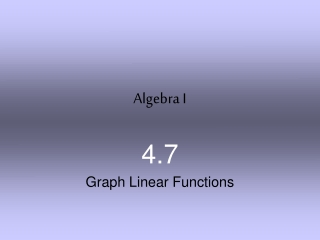DownloadDownload PresentationAlgebra I

# Algebra I

Télécharger la présentation## Algebra I

- - - - - - - - - - - - - - - - - - - - - - - - - - - E N D - - - - - - - - - - - - - - - - - - - - - - - - - - -
##### Presentation Transcript

1. Algebra I 4.7 Graph Linear Functions

2. f (x) 3x– 15 = (–3)3(– 3) – 15 = f 24 = ANSWER D B The correct answer is A. A C EXAMPLE 1

3. f(x) 6. = f(x) = 2x – 10 62x – 10 = 8 x = GUIDED PRACTICE 1. Evaluate the function h(x)=– 7x when x =7. h(7) = – 49 2. For the function f(x)2x–10, find the value of xso that =

4. EXAMPLE 3 The gray wolf population in central Idaho was monitored over several years for a project aimed at boosting the number of wolves. The number of wolves can be modeled by the function f(x) = 37x + 7 wherexis the number of years since1995. Graph the function and identify its domain and range. GRAY WOLF

5. > > = = The domain of the function is x 0. From the graph or table, you can see that the range of the function is f(x) 7. Graph a function EXAMPLE 3 To graph the function, make a table.

6. x f(x) 37(0) + 7 = 7 0 37(1) + 7 = 44 1 37(2) + 7 = 81 2 37(3) + 7 = 118 3 37(4) + 7 = 155 4 2.WOLF POPULATIONUse the model from Example 3 to find the value of xso that f(x) = 155. Explain what the solution means in this situation. SOLUTION 4 years after 1995, the wolf population will be 155.

7. Graph the function. Compare the graph with the graph of f (x) x. = a. g(x) = x + 3 EXAMPLE 4 b. h(x) = 2x Because the slope of the graph of h is greater than the slope of the graph of f, the graph of h rises faster from left to right. The y-intercept for both graphs is 0, so both lines pass through the origin. Because the graphs of g and f have the same slope, m = 1, the lines are parallel. Also, the y- intercept of the graph of g is 3 more than the y-intercept of the graph of f.

8. 3. Graph h(x) = – 3x. Compare the graph with the graph of f (x) = x. GUIDED PRACTICE Since the slope of the graph of h is negative the graph of h falls from left to right. The y-intercept for both graphs is 0, so both lines pass through the origin.

9. EXAMPLE 5 CABLE A cable company charges new customers \$40 for installation and \$60 per month for its service. The cost to the customer is given by the function f(x) = 60x +40 where x is the number of months of service. To attract new customers, the cable company reduces the installation fee to \$5. A function for the cost with the reduced installation fee is g(x) = 60x + 5. Graph both functions. How is the graph of g related to the graph of f ?

10. EXAMPLE 5 The graphs of both functions are shown. Both functions have a slope of 60, so they are parallel. The y-intercept of the graph of g is 35 less than the graph of f. So, the graph of g is a vertical translation of the graph of f.